Information for Maple assignment 2, spring 2006

This link describes the second Maple assignment.
Each student will get individual data by e-mail for the assignment.
The assignment is due Wednesday, February 15.

Here is some help for the second Maple assignment. Please note that some of the commands discussed here require loading the plots package:
>with(plots);
Others require the VectorCalculus package:
>with(VectorCalculus);
I repeat what I wrote in the last set of instructions:
You are allowed and, indeed, encouraged to copy and modify the commands discussed here.

Pictures of a "molecule"
Here's a description by formulas of the "molecule" or curve under investigation in these pictures:
x=5cos(2t)+5sin(t) and y=2cos(2t)+4cos(t) and z=sin(3t)+4sin(t)
I'm beginning with this curve because it is fairly simple. I'll try to show more complicated curves later. This curve is a closed curve on the interval [0,2Pi] because the formulas are all combinations of sines and cosines with integers multiplying the parameter, t.

This first picture shows the result of the command
>spacecurve(Q(t),t=0..2*Pi);
I had previously defined Q(t) with a command that looked like this:
>Q:=t-><5*cos(2*t)+5*sin(t),2*cos(2*t)+4*cos(t),sin(3*t)+4*sin(t)>
The result is the picture shown below.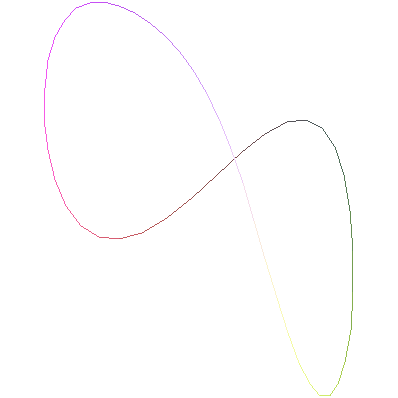I don't think this picture is very useful. So I changed the command to
>spacecurve(Q(t),t=0..2*Pi,thickness=2,color=black,axes=normal,
labels=[x,y,z],numpoints=150);

All commands should be typed on one line.
They're on several lines here to control the web page's width.

I used "options" which I specified after some experimentation. The thickness and color made the curve easier to see. The labels on the axes are also useful if the image is rotated. The numpoints option increases the number of sampling points, because mostly what Maple does is plot points (the default, I think, is 50) and then connect these points with straight line segments. If you want to play, see what happens with numpoints=20 or numpoints=10. You'll get very polygonal (corners, line segments) approximations to the curve. The increases specification for numpoints made the curves I experimented with seem less polygonal.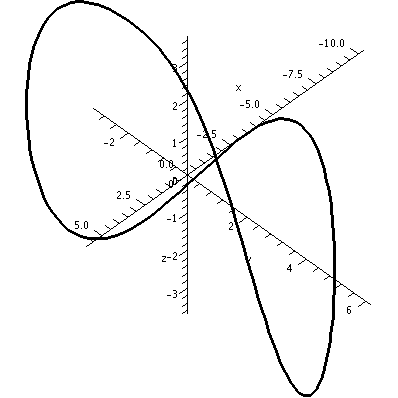Certainly the view shown is much nicer, and has some readable quantitative information (on the axes). It is, however, a bit deceptive. The picture seems to show the curve intersecting itself. In fact, this particular curve does not have any self-intersections. This is what's called a simple closed curve. If you right-click on a Maple picture, the Manipulator button allows you to rotate or scale or pan. So you can get many different views. Here is a view which shows the same curve from a different angle, and from a bit "farther" away. Apparently the curve does not intersect itself!

Warning There are curves which do not intersect themselves, but have the irritating (?) property that every two dimensional view shows intersections!We can try various views to get the size of the curve. Two images are shown below.
The image on the left has the z-axis sticking directly out from the viewing plane.
The image on the right has the y-axis sticking directly out from the viewing plane. Therefore it shows a picture of the curve squooshed (??) down on the xz-plane. Unfortunately (?) due to carelessness, the z-axis is inverted from its standard presentation (positive is down in this picture and negative is up).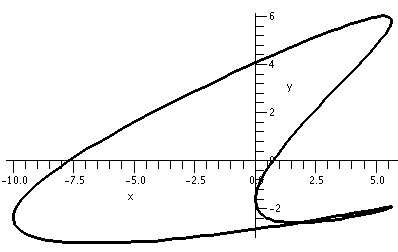From these pictures I can get a rough idea of the dimensions of this curve. Apparently the curve fits inside a box with -10<x<6 and -3.5<y<6 and -4<z<4. One advantage of the two views is that they provide a rough way to check, because they both show the x-axis.How long is this curve? The parameter travels from 0 to 2Pi. We need to differentiate the position vector, and then find its length or magnitude (this is the speed). The function which computes the vector's length in the VectorCalculus package is called Norm. So here is the speed computation:
> dsdt:=Norm(diff(Q(t),t));
dsdt:=((-10*sin(2*t)+5*cos(t))^2+(-4*sin(2*t)-4*sin(t))^2+(3*cos(3*t)+4*cos(t))^2)^(1/2)
If we want the length, we need to integrate this from t=0 to t=2Pi. Here is what happened when I tried a "direct" computation on my computer at home and also requested, before and after the computation, the amount of CPU time used.
> time();
.222
> int(dsdt,t=0..2*Pi);
int(((-10*sin(2*t)+5*cos(t))^2+(-4*sin(2*t)-4*sin(t))^2+(3*cos(3*t)+4*cos(t))^2)^(1/2),
t=0..2*Pi); > time(); 12.114
Maple returned the original integral, not evaluated, although the program did "think" about it for almost a dozen CPU seconds! This tells me that Maple was unable to evaluate this integral symbolically, which is not surprising -- "most" functions defined by formulas can't be antidifferentiated in terms of familiar functions.

Maple needs to be "convinced" to evaluate this integral approximately. Here's how to do that:

> evalf(Int(dsdt,t=0..2*Pi));
56.13189562
Please note the capital I in the integrate command. This is an important difference which you can read about on a help page if you want. The numerical computation of the integral took less than a hundredth (.01) of a second. This curve is about 56 units long.This is not Maple's Norm.
(He is a master carpenter on a TV show about
home renovations called This Old House.)
Maple also computes curvature as one of the procedures in the VectorCalculus package. So here is the curvature of this curve:
> Curvature(Q(t),t);
((-1/2/(-200*sin(t)*cos(t)^2+144*cos(t)^6-584*cos(t)^4+498*cos(t)^2+64*cos(t)+
16-64*cos(t)^3)^(3/2)*(-10*sin(2*t)+5*cos(t))*(-200*cos(t)^3+400*sin(t)^2*cos(
t)-864*cos(t)^5*sin(t)+2336*cos(t)^3*sin(t)-996*sin(t)*cos(t)-64*sin(t)+192*
sin(t)*cos(t)^2)+1/(-200*sin(t)*cos(t)^2+144*cos(t)^6-584*cos(t)^4+498*cos(t)^
2+64*cos(t)+16-64*cos(t)^3)^(1/2)*(-20*cos(2*t)-5*sin(t)))^2+(-1/2/(-200*sin(t
)*cos(t)^2+144*cos(t)^6-584*cos(t)^4+498*cos(t)^2+64*cos(t)+16-64*cos(t)^3)^(3
/2)*(-4*sin(2*t)-4*sin(t))*(-200*cos(t)^3+400*sin(t)^2*cos(t)-864*cos(t)^5*sin
(t)+2336*cos(t)^3*sin(t)-996*sin(t)*cos(t)-64*sin(t)+192*sin(t)*cos(t)^2)+1/(-\
200*sin(t)*cos(t)^2+144*cos(t)^6-584*cos(t)^4+498*cos(t)^2+64*cos(t)+16-64*cos
(t)^3)^(1/2)*(-8*cos(2*t)-4*cos(t)))^2+(-1/2/(-200*sin(t)*cos(t)^2+144*cos(t)^
6-584*cos(t)^4+498*cos(t)^2+64*cos(t)+16-64*cos(t)^3)^(3/2)*(3*cos(3*t)+4*cos(
t))*(-200*cos(t)^3+400*sin(t)^2*cos(t)-864*cos(t)^5*sin(t)+2336*cos(t)^3*sin(t
)-996*sin(t)*cos(t)-64*sin(t)+192*sin(t)*cos(t)^2)+1/(-200*sin(t)*cos(t)^2+144
*cos(t)^6-584*cos(t)^4+498*cos(t)^2+64*cos(t)+16-64*cos(t)^3)^(1/2)*(-9*sin(3*
t)-4*sin(t)))^2)^(1/2)/(-200*sin(t)*cos(t)^2+144*cos(t)^6-584*cos(t)^4+498*cos
(t)^2+64*cos(t)+16-64*cos(t)^3)^(1/2)
This is certainly an example of why people use : instead of ;. The colon doesn't display its output. I think that knowledge of the specific formula of the curvature is not likely to be useful.

But I can compute the curvature at, for example, t=2:

> evalf(subs(t=2,Curvature(Q(t),t)));
.2586332282
The subs command substitutes 2 for t in the result of the Curvature(Q(t),t) command, and then evalf asks for a digital approximationBBB.

Below is the result of the command
plot(Curvature(Q(t),t),t=0..2*Pi,thickness=2);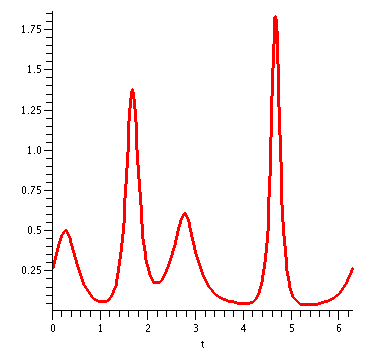I can use this graph to locate (approximately) the curviest (most curvy?) point on the molecule. If you position your mouse image on the graph, and left click, the cursor position relative to the graph is shown to the right, above the window. In this case, the coordinates that I see for The top are 4.69 and 1.81. So the highest curvature in this curve is about 1.81 and this occurs when the parameter t is about 4.69.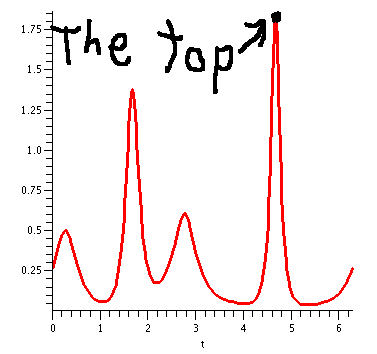Where is the curviest point? We must substitute the value of the parameter, t=4.69, into the curve function. In the command line interface, here's how I'd find it:
>evalf(subs(t=4.69,Q(t)));
Vector[column](3,{(1) = -9.993735059, (2) =
-2.087543709, (3) = -3.001252360}, datatype = anything,storage =
rectangular,order = Fortran_order,shape = [])
The same command in the GUI (graphical user interface) gives a cleaner answer, but the answer in both is about (-9.994,-2.086,-3.001).

Now I'll try to locate the point, the most curvy point, on the graph. I'll create two pictures, and then display them together:

> MCP:=plot3d([-9.994,-2.086,-3.001],x=-10..5,y=-5..5,labels=[x,y,z],
symbol=box,axes=normal,color=blue,style=point): > CURVE:=spacecurve(Q(t),t=0..2*Pi,thickness=2,color=black,axes=normal,
labels=[x,y,z],numpoints=150): > display({MCP,CURVE})
The picture on the left was displayed. I was confused. The little blue box is supposed to be the MCP ("most curvy point"). But what's near the MCP candidate didn't look very curvy compared to other parts of the curve. But then I "adjusted" the image to Scaling=Constrained and the result was the picture on the right. I was happier.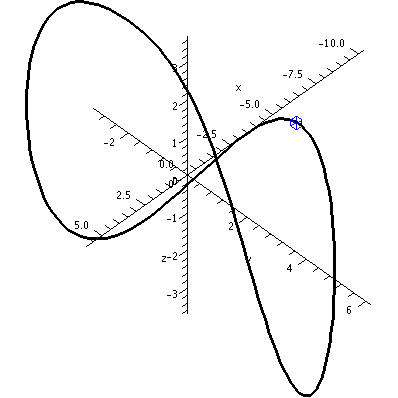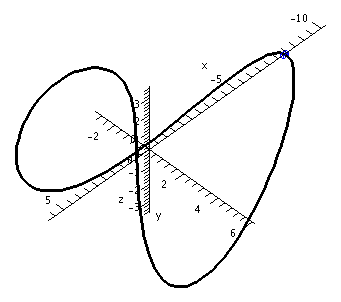I chose the example that I analyzed in detail above because it is fairly simple. Students will get randomly selected curves. They'll also get the curvature of their random curve at t=2, so that they can at least check initially that they've entered the functions correctly.

Some strange things can happen. Let me show you a few of them.

Weirder pictures
Below is a plot of curvature for a curve which isn't as nice. The curvature has an enormous thin peak. Experiments seem to show that such behavior is common.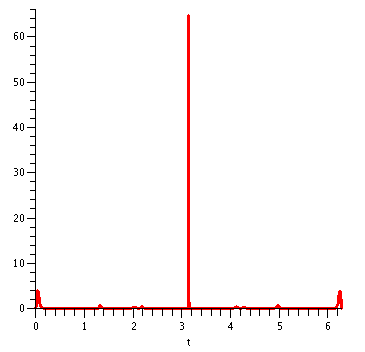Below is the curve that whose curvature graph is above. On the left is an constrained view, and on the right, an unconstrained view. There is very high curvature in a tiny piece of the curve.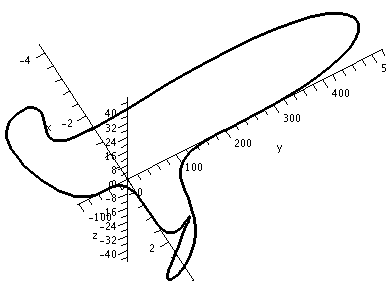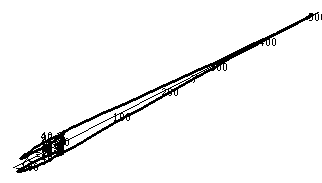Below are three pictures of another curve. I emphasize that these are all pictures of the same curve. This curve does not intersect itself, but every two dimensional view does seem to show an intersection. So detecting intersections by "just looking" may be a bit difficult. Some examination of the images is necessary.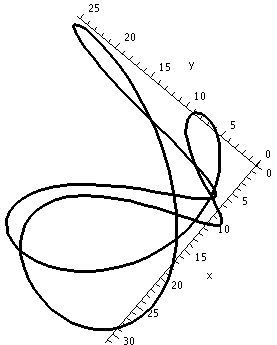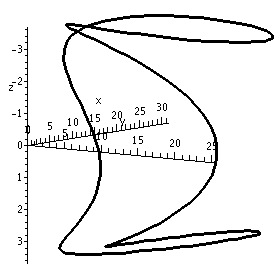Here are two pictures of yet another closed curve. This closed curve does have two self-intersections. I am not sure how to persuade people of this without much numerical work or many carefully chosen pictures. If you have suggestions, please let me know.I am very curious to learn if this homework assignment will lead to any bizarre pictures and numbers. Please tell me if you think you have found some.
I thank Ms. L. Pudwell for several useful conversations about this material.

Note After experiments with some students, I am very glad that torsion was not mentioned in this assignment! The torsion computations seem to be quite elaborate, taking large amounts of memory and computational time. I think we could have "frozen" huge chunks of university computer resources with any general requests for torsion. I know that I caused my computers at home and at school to be very unhappy by asking for the torsion of some students' curves.

Maintained by greenfie@math.rutgers.edu and last modified 2/5/2006.